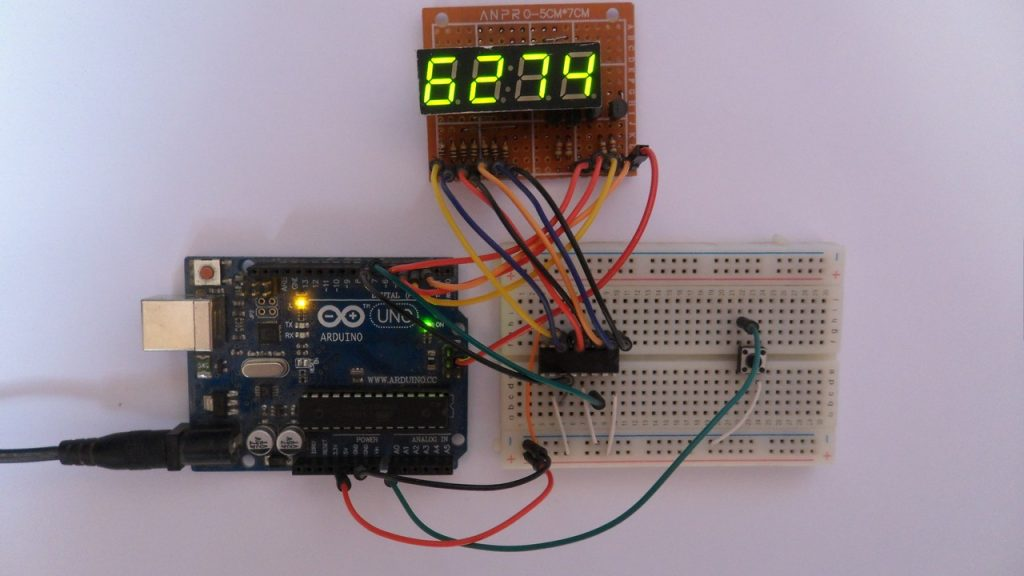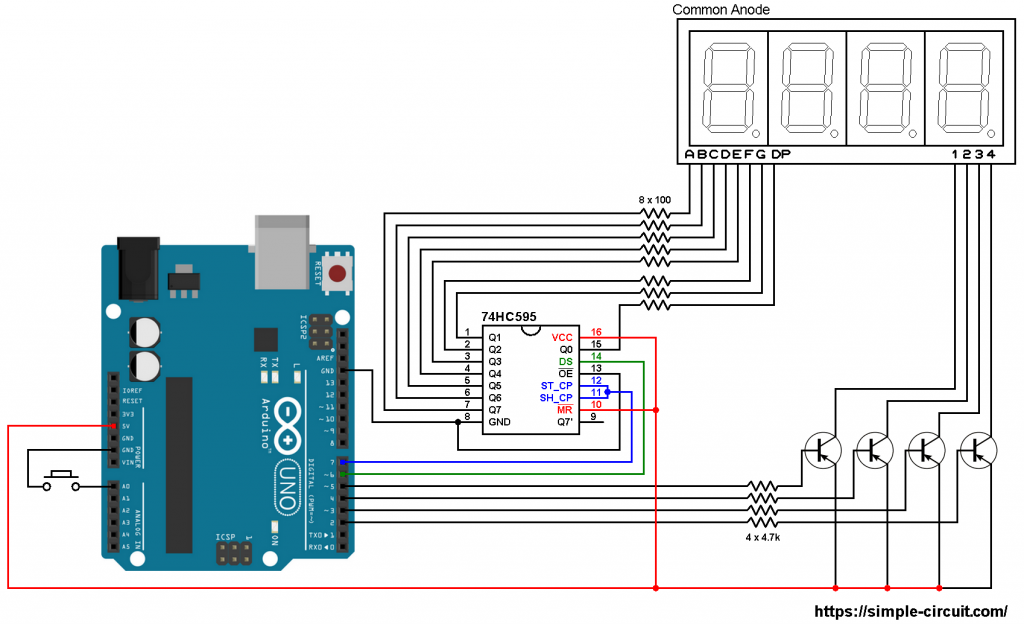# 7-Segment display with 74HC595 shift register | Arduino Projects

Adding a serial-in parallel-out shift register such as the popular one 74HC595 to a 7-segment display will reduce number of pins required to drive it (the display).
Basically the 7-segment display requires 9 pins: 8 segment pins (A, B, C, D, E, F, G and DP) + common pin. By connecting all the segment pins to a shift register, the required number of pins becomes just 3: clock pin and data pin (for the shift register) + common pin.
So for a 4-digit 7-segment display we need just 6 pins: clock, data and 4 common pins (each digit has its individual common pin).

This topic shows how to build a simple digital counter using Arduino, common anode 7-segment display with 4 digits and 74HC595 shift register.

To see how to interface Arduino with 7-segment display (without shift register) visit the following post:
Interfacing Arduino with 7-segment display | 4-Digit counter exampleHardware Required:

• Arduino UNO board
• 4-Digit common anode 7-segment display
• 74HC595 shift register   —->   datasheet
• 4 x PNP transistor (2SA1015, 2S9015, 2N3906 …)
• 8 x 100 ohm resistor
• 4 x 4.7k ohm resistor
• Push button
• Jumper wires

Arduino 7-Segment display with 74HC595 shift register circuit:
The image below shows our example circuit schematic diagram.As shown in the circuit diagram above, all segment pins are connected to the 74HC595 output pins, each one through 100 ohm resistor, where:
Segment A … G are connected to 74HC595 pin Q7 … Q1 respectively and segment DP is connected to pin Q0.
The data pin of the 74HC595 shift register is named DS (#14) and it is connected to Arduino pin 6.
ST_CP (or RCLK) and SH_CP (or SRCLK) are connected together which then connected to Arduino pin 7. This is the clock pin.

Since the display has 4 digits there’re 4 common pins: 1 (most left), 2, 3 and 4. Each common pin is connected to collector terminal of one transistor. Emitter terminals of the 4 transistors are connected to +5V which comes from the Arduino board. Base terminals of the four transistors are connected to Arduino through 4.7k resistors.

The 4 transistors are of the same type (PNP).

The push button which is connected to Arduino analog pin 0 (A0) is used to increment the displayed number.

7-Segment display with 74HC595 shift register code:
The Arduino code below doesn’t use any library for the 7-segment display.

The button connection is defined in the code as:

Shift register clock pin and data pin are defined as:

The display needs to be refreshed periodically, for that I used Timer1 module interrupt with the following configuration:

With Timer1 prescaler = 1, we’ve an interrupt every 4096 microseconds. That means every digit is displayed for 4096 us. { 4096 us = 65536 / (16 * prescaler) }
Note that Timer1 module is 16-bit timer and Arduino clock frequency is 16MHz.

Functions used in the code:
ISR(TIMER1_OVF_vect) : is Timer1 interrupt function, when the microcontroller interrupted by Timer1 it will directly execute this ‘function’.

void disp(byte number, bool dec_point = 0) : this function is for printing data on the 7-segment display, it prints the variable number which may vary between 0 and 9. The variable dec_point decides whether the DP will be printed or not, the default value is 0 (don’t print), if dec_point = 1 the DP segment will be ON.

void disp_off() : this function turns off the whole display.

I used Arduino shiftOut function (built-in) to send data serially to the 74HC595 shift register.

Full Arduino code:

The result of this example should be the same as the one shown in the following video (4-digit counter without shift register):

1.Prathamesh Vengurlekar says:

can u give us common cathode clock values 7 values from 0 to 9 case of this project please

2.IBRAHIM says:

can u give us common cathode clock values 7 values from 0 to 9 case of this project please ????
3.ximikas says:
4.ZAGO1819 says: